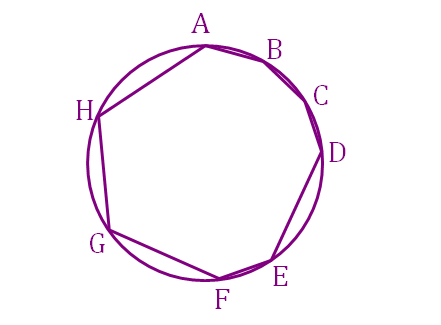# That's an Ugly Looking Octagon

Geometry Level 4As shown in the diagram above, $ABCDEFGH$ is an inscribed octagon where $AB=BC=CD=EF=1$ and $DE=FG=GH=HA=2$.

If the area of the octagon can be expressed as $a+b\sqrt c,$ where $a, b, c$ are all integers and $c$ is square-free, then find the value of $a+b+c$.

×

Problem Loading...

Note Loading...

Set Loading...